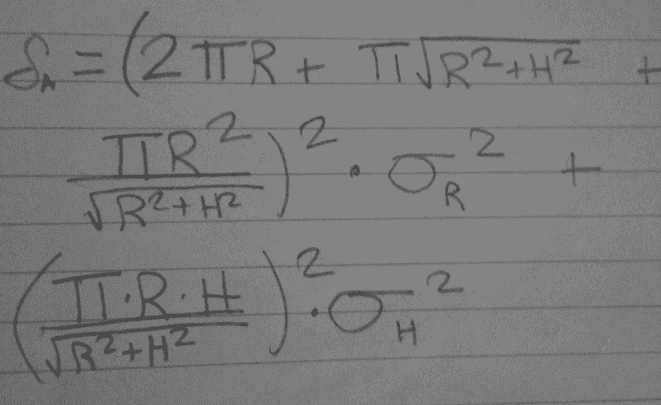# Uncertainty homework help

• Panphobia
In summary, the uncertainty in the area of a right circular cone with radius 10.0 cm and height 15.0 cm is +/- 14.6 cm².

#### Panphobia

Uncertainty partial derivatives

## Homework Statement

1 a) Give expressions for the uncertainty in both the area and volume of a right circular cone with radius R and height H and side length S. Assume that the radius has uncertainty σR and the height has an uncertainty σH. <----First part that I got.

b) For a radius R = 10.0 ± 0.1 cm and height H = 15.0 ± 0.2 cm, calculator the absolute uncertainty on both the area and the volume.

## Homework Equations

Surface area A = ∏*R^2 + (PI)*S*R
Volume V = (1/3)*∏*R^2*H
(S = sqrt(R^2 + H^2))

## The Attempt at a Solution

Ok so my attempt at solving this question was as follows

I tried to figure out the partial derivative with respect to the radius, and with respect to the height. When you get those the formula is

I was having trouble using the latex math equations thing so I just took a picture and here it is...
if it is unclear sigma and delta mean the same thing.now I plugged in the values R = 10.0 ± 0.1 cm and height H = 15.0 ± 0.2 cm, and I got some absurdly high values, am I right, or did I do something wrong in my partial derivatives?

Last edited:
Your equations are correct, except that their left-hand sides must have squares of the deltas.

So for the volume the uncertainty is + or - 37.8, and the uncertainty for area is + or - 13.7? Did I plug them in correctly now?

I get the same for the volume, but 14.6 for the area. I ended up using a spreadsheet to compute things after a few blunders :)

I'm seeing ~ 9.1 for the area (just saying...).

Oh my...which one is right. I don't knowww

Since we all seem to agree on the volume, consider it correct. For area, I suggest you post the value you get for each term in the equation, so that we could then cross-check.

I get 187.4 for the first section until sigma r, then 27.33 for the rest. Then I add them and take the square root and get 14.6.

I get the same results. Perhaps gneill could comment on his numbers.

voko said:
I get the same results. Perhaps gneill could comment on his numbers.

Okay, I see that the base surface area is to be included and not just the lateral area of the right circular cone. I didn't notice that before, so that explains a source of difference. My bad.

Designating the differentials of the Area as DAr and DAh, and dropping the units for the calculations so that r = 10.0, h = 15.0, then I find (keeping extra decimal places for intermediate calculations):

DAr = 136.894
DAh = 26.140

and so
$$δA = \sqrt{DA_r^2 δr^2 + DA_h^2 δh^2} = 14.6$$